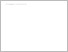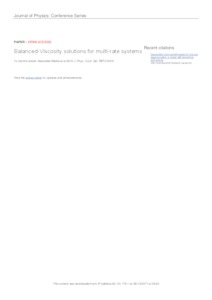Repository: Freie Universität Berlin, Math Department

# Balanced-Viscosity solutions for multi-rate systems

Mielke, A. and Rossi, R. and Savaré, G. (2016) Balanced-Viscosity solutions for multi-rate systems. Journal of Physics: Conference Series, 727 . pp. 1-27.Preview

1MB

Official URL: http://dx.doi.org/10.1088/1742-6596/727/1/012010

## Abstract

Several mechanical systems are modeled by the static momentum balance for the displacement u coupled with a rate-independent flow rule for some internal variable z. We consider a class of abstract systems of ODEs which have the same structure, albeit in a finite-dimensional setting, and regularize both the static equation and the rate-independent flow rule by adding viscous dissipation terms with coeficients \epsilon^\alpha and \epsilon, where 0 < \epsilon << 1 and \alpha > 0 is a fixed parameter. Therefore for \alpha\neq 1 u and z have different relaxation rates.<br /> We address the vanishing-viscosity analysis as \epsilon\downarrow 0 of the viscous system. We prove that, up to a subsequence, (reparameterized) viscous solutions converge to a parameterized curve yielding a Balanced Viscosity solution to the original rate-independent system, and providing an accurate description of the system behavior at jumps. We also give a reformulation of the notion of Balanced Viscosity solution in terms of a system of subdifferential inclusions, showing that the viscosity in u and the one in z are involved in the jump dynamics in different ways, according to whether \alpha > 1, \alpha = 1, and \alpha\in (0, 1).

Item Type: Article Mathematical and Computer Sciences > Mathematics > Numerical Analysis Department of Mathematics and Computer Science > Institute of Mathematics 2152 Silvia Hoemke 07 Dec 2017 17:50 08 Dec 2017 14:21

Repository Staff Only: item control page